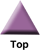### Home

Laboratory
Studies

Recordkeeping, Writing,
& Data Analysis

Laboratory
Methods

Overview
Microscope studies

Flagella experiment
Laboratory math
Blood fractionation
Gel electrophoresis
Protein gel analysis
Mitochondria
Concepts/ theory
Overview
Keeping a lab notebook
Writing research papers
Dimensions & units
Using figures (graphs)
Examples of graphs
Experimental error
Representing error
Applying statistics
Overview
Principles of microscopy

Solutions & dilutions
Protein assays
Spectrophotometry
Fractionation & centrifugation

# Volumes, Amounts, and Concentrations

The common language of scientists uses units of measurement that are recognized in labs all over the world, appropriately called the International System of Units. The seven SI (for Systeme Internationale) base units are all defined in terms of well established physical quantities or standards. Other units of measure were derived from the SI base units and are called SI-derived units. In solution making we work with SI or SI-derived units for volumes, amounts, and concentrations.

Volume describes the space taken up by something. Since space is three-dimensional, a unit of volume is the cube of a unit of length, such as centimeters, feet, inches, or meters. An SI-derived unit for volume is the liter, defined as 1000 cubic centimeters. One cubic centimeter, or one thousandth liter, is a milliliter. We use prefixes in order to simplify quantitative expressions of volume, amount, and concentration. For example, we might find it convenient to describe a volume in microliters (one millionth liter) or deciliters (1 tenth liter).

The SI base unit for an amount of substance is the mole. The mole and its use in defining solutions will be discussed later. A less specific unit with which to describe an amount of substance is a unit of mass. The SI base unit for mass is the kilogram, which is 1000 grams. We may describe quantities using milligrams (one thousandth of a gram), micrograms (one millionth of a gram), or perhaps even nanograms (one billionth of a gram).

Concentration refers to the amount of substance within a specified volume. Solutions are defined by the type of solute or solutes, the type of solvent, the concentration of each solute, and often (for aqueous solutions), pH. Suspensions can also be defined as the amount of a minor component per unit total volume (volume of minor components plus volume of solvent). The concentration of a suspension can be described as the number of particles per unit volume, as is often the case for cell suspensions.

When you settle on units with which to describe quantities, you should select an appropriate prefix so that the expressions are simplified as much as possible. For example, if most of the concentrations you report are less than a thousandth of a mole per liter, it is much preferable to report them using millimoles per liter. The quantity 0.00045M is the same as the quantity 0.45 mM. You should reduce large numbers to smaller ones if you can. For example, 48,000 milligrams (mg) is the same as 48 grams.

## Prefixes (International System of Units)

A student of the natural sciences really should be comfortable using prefixes to modify units.  You should be familiar with at least some of the prefixes, from prior course experience.

 Prefix Factorbase 10 Symbol Prefix Factorbase 10 Symbol deci -1 d deca 1 da centi -2 c hecto 2 h milli -3 m kilo 3 k micro -6 µ mega 6 M nano -9 n giga 9 G pico -12 p tera 12 T femto -15 f peta 15 P atto -18 a exa 18 E